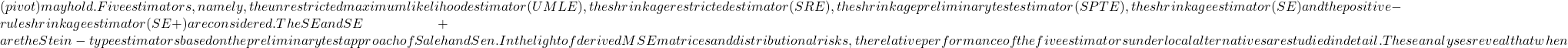# Some improved estimators in logistic regression model

The problem of estimating the parameters of logistic regression model is considered
when it is known from extraneous sources that theH_0:\beta_0=\ldots, =\beta_{k-1}=\beta^0k  \geq 3k \leq 2\$ it is advisable to use the
preliminary test estimator (PTE).

Fulltext